# Informatics1-2019/Lab09

(Változatok közti eltérés)

# MatLab exercises

## Linear Equations

Determine the existence of a solution and all the solutions, if any!

### a

 x + 5y = 1
2x + 4y = 2


### b

 x + 5y = 1
2x + 4y = 2
5x - 6y = -1


### c

 x + 2y + 5z = 1
5x + 4y + 6z = 2


Implement a general, linear equation solver function!

## Tricky matrices

Generate the following matrix with a command:

    1     2     3     4     5     6     7     8     9    10
2     3     4     5     6     7     8     9    10    11
3     4     5     6     7     8     9    10    11    12
4     5     6     7     8     9    10    11    12    13
5     6     7     8     9    10    11    12    13    14
6     7     8     9    10    11    12    13    14    15
7     8     9    10    11    12    13    14    15    16
8     9    10    11    12    13    14    15    16    17
9    10    11    12    13    14    15    16    17    18
10    11    12    13    14    15    16    17    18    19


### Multiplication table

Generate a modulo-7 multiplication table:

    0     0     0     0     0     0     0
0     1     2     3     4     5     6
0     2     4     6     1     3     5
0     3     6     2     5     1     4
0     4     1     5     2     6     3
0     5     3     1     6     4     2
0     6     5     4     3     2     1


### generalization

Write a function with one argument (n) that gives the modulo-n multiplication table, if n is a prime. Otherwise return an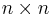$n\times n$ matrix full of zeros.

isprime


## Reductions

### a

Write a function that determines the centroid (center of mass) of a list of points in 3D. The function should have one argument: a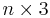$n\times3$ matrix where each line is a 3D (row) vector. The output should be a row vector of length 3, the centroid.

### b

Write a function that calculates the mean of absolute values of the entries in a matrix.

### c

Write a function that calculates the maximum row-wise absolute sum of a matrix.

 M = maxi ∑ | Ai,j | j

### d

Write a function that calculates the maximum column-wise absolute sum of a matrix.

 m = maxj ∑ | Ai,j | i

### e

Write a function that calculates the square-sum of each slice in a 3-dimensional array and returns the maximum of those values. For one matrix, the square-sum is: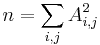$n = \sum_{i,j} A_{i,j}^2$

The greatest square-sum is: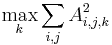$\max_k \sum_{i,j} A_{i,j,k}^2$

## Plot

### a

Write an absolute value function (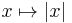$x\mapsto|x|$) and plot it.

### b

Plot the 3D spiral from the lecture note. How can you choose the axes where the spiral turns around? Can you modify the number of turns to make it twist more rapidly?

### c

Plot the$(x,y) \mapsto x+y$ function on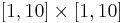$[1,10]\times[1,10]$

### d

Plot the$(x,y) \mapsto gcd(x,y)$ function on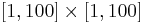$[1,100]\times[1,100]$ (only integer points).

## Integral

Write a function with 4 arguments: a function and three numbers.

 int(f, a, b, dx)


Calculate the approximation of the integral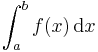$\int_a^b f(x)\,\mathrm{d}x$ with trapezoidal rule.

## Differentiation

Let x and f be two vectors with the same length where (xi,f(xi)) means corresponding x and f(x) values. For example:

x = 0:0.1:6;
f = sin(x);


Plot the function and its numeric derivative.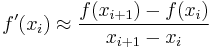$f'(x_i) \approx \frac{f(x_{i+1})-f(x_{i})}{x_{i+1}-x_{i}}$

Try out some finite difference methods